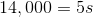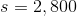## Example Questions

### Example Question #1 : How To Find The Length Of The Side Of A Pentagon

The perimeter of a regular pentagon is. What is the length of one side?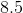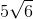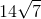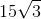Explanation:

A problem like this is very easy. A regular pentagon just looks like:All of its sides are equal. Therefore, you know that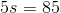, whereis the length of one side. Solving for, you get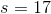.

### Example Question #2 : How To Find The Length Of The Side Of A Pentagon

If the perimeter of a given regular pentagon is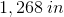. What is the length of one side of this regular pentagon in inches?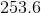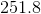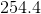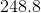Explanation:

Use the formula for perimeter to solve for the side length of the regular pentagon: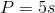Whereis the perimeter andis the length of a side.

In this case: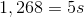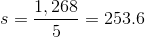### Example Question #3 : How To Find The Length Of The Side Of A Pentagon

Find the length of each side for regular pentagon with a perimeter of.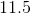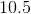Explanation:

Use the formula for perimeter to solve for the side length: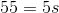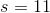### Example Question #4 : How To Find The Length Of The Side Of A Pentagon

Find the length of one side for a regular pentagon with a perimeter of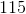.Explanation:

Use the formula for perimeter to solve for the side length: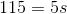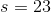### Example Question #5 : How To Find The Length Of The Side Of A Pentagon

Find the length of each side for a regular pentagon with a perimeter of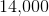.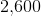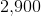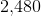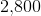Explanation:

Use the formula for perimeter to solve for the side length: